##### Microeconomics For Dummies - UK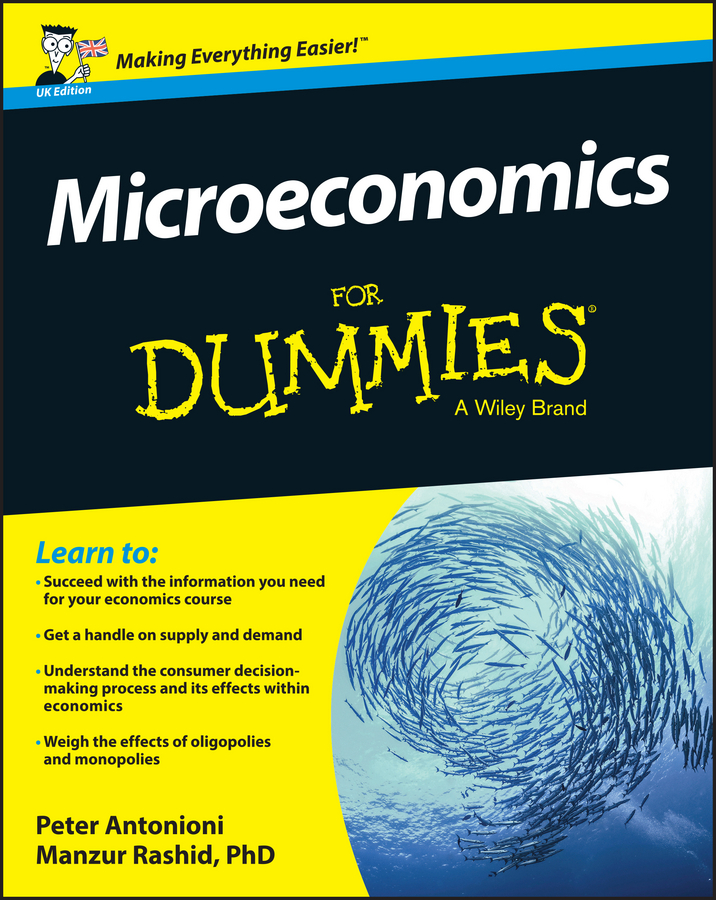Economic profit is defined as the difference between total revenue and the explicit plus implicit costs of production. It’s the same as profit. Economic profit per unit equals price minus average total cost, or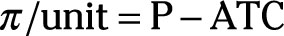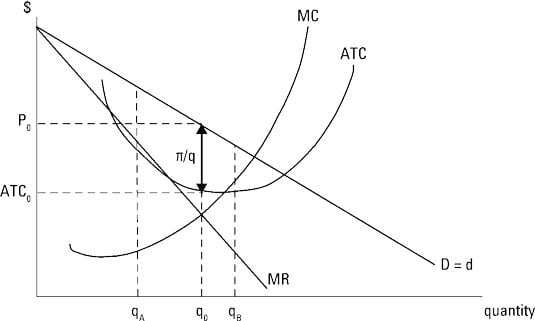In this illustration, economic profit per unit is illustrated by the double-headed arrow labeled ð/q.

Total profit equals profit per unit multiplied by the number of units sold, or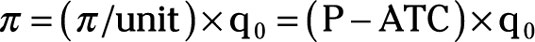Using the same information in the previous example, the monopolist’s demand curve is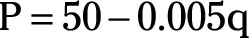And the monopolist’s total cost equation is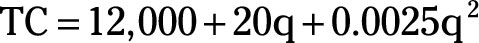Given this information, the profit-maximizing quantity is 2,000 units at a price of \$40 per unit.

In order to determine the monopolist’s economic profit per unit and total profit, you take the following steps:

1. Determine the average total cost equation by dividing the total cost equation by the quantity of output q.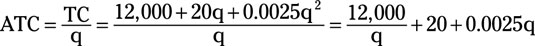2. Substitute q equals 2,000 in order to determine average total cost at the profit-maximizing quantity of output.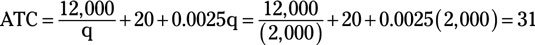Thus, the average total cost is \$31 at the profit-maximizing quantity of 2,000 units.

3. Calculate profit per unit.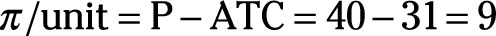Profit per unit equals \$9.

4. Determine total profit by multiplying profit per unit by the profit-maximizing quantity of output.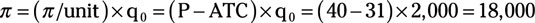Total profit equals \$18,000.

Don’t confuse maximizing total profit with maximizing profit per unit. You’re willing to accept less profit per unit if you sell a lot more units. For example, if your profit per unit was \$11 and you sold 1,265 units of output, your total profit equals \$13,915.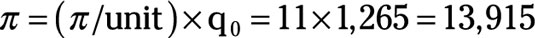On the other hand, if your profit per unit is only \$9 but you’re now able to sell 2,000 units because you charge a lower price, your total profit equals \$18,000.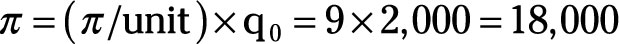Remember, your goal is always to maximize total profit.